# Consider light with a wavelength of 519 nm is passing through a diffraction grating with 1208...

Consider light with a wavelength of 519 nm is passing through a diffraction grating with 1208 lines per cm. At what distance from the central bright maximum will the 1st order maximum be seen on a screen placed 1.20 m from the diffraction grating. Give your answer in centimeters (cm) with 3 significant figures please. :)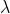= wavelength = 519 nm = 519 x 10-9 m

d = slit width = 0.01/1208 = 8.3 x 10-6 m

D = screen distance = 1.20 m

n = 1

y = distance from central bright maximum

using the equation

y = nD/d

y = 1 (1.20) (519 x 10-9) /(8.3 x 10-6) = 0.075 m = 7.50 cm

#### Earn Coin

Coins can be redeemed for fabulous gifts.

Similar Homework Help Questions
• ### QUESTION 7 Light of 430 nm passing through a diffraction grating with a separation, d=1.5 x...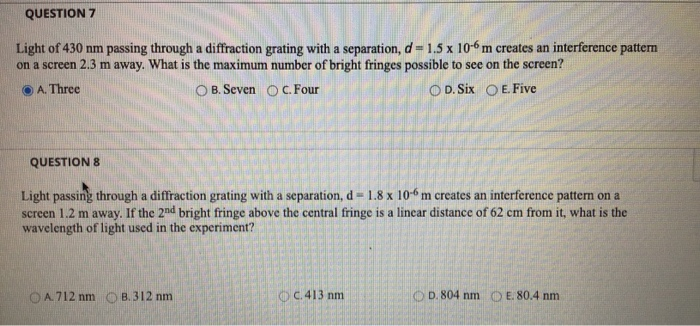QUESTION 7 Light of 430 nm passing through a diffraction grating with a separation, d=1.5 x 10-6 m creates an interference pattern on a screen 2.3 m away. What is the maximum number of bright fringes possible to see on the screen? A. Three OB. Seven C. Four O D. Six O E. Five QUESTIONS Light passing through a diffraction grating with a separation, d = 1.8 x 10m creates an interference pattern on a screen 1.2 m away. If...

• ### 4oonn Problem #3. A light of wave length 400 Nm falls on a DIFFRACTION GRATING having...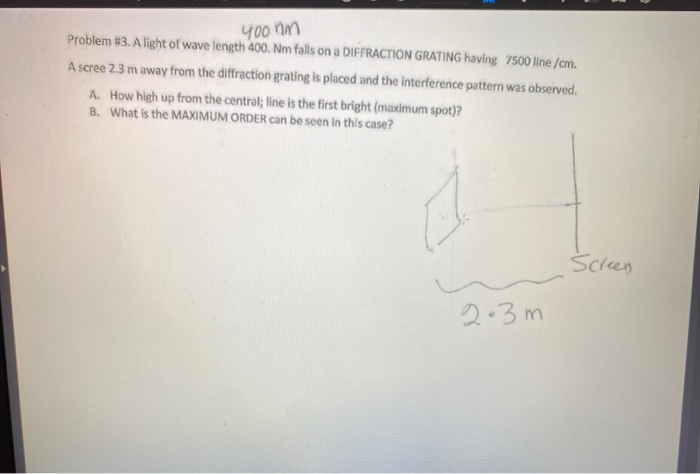4oonn Problem #3. A light of wave length 400 Nm falls on a DIFFRACTION GRATING having 7500 line /cm. A scree 2.3 m away from the diffraction grating is placed and the interference pattern was observed. A. How high up from the central; line is the first bright (maximum spot)? B. What is the MAXIMUM ORDER can be seen in this case? Screen 2.3m

• ### 4oonin Problem #3. A light of wave length 400. Nm falls on a DIFFRACTION GRATING having...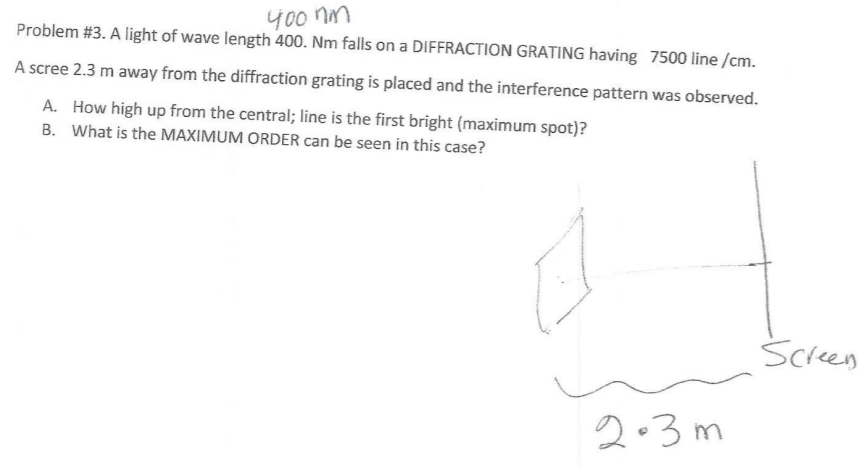4oonin Problem #3. A light of wave length 400. Nm falls on a DIFFRACTION GRATING having 7500 line /cm. A scree 2.3 m away from the diffraction grating is placed and the interference pattern was observed. A. How high up from the central; line is the first bright (maximum spot)? B. What is the MAXIMUM ORDER can be seen in this case? Screen 2.3m

• ### A diffraction grating with 600 lines/mm is illuminated with light of wavelength 510 nm. A very...

A diffraction grating with 600 lines/mm is illuminated with light of wavelength 510 nm. A very wide viewing screen is 4.2 m behind the grating. Part A What is the distance between the two m = 1 bright fringes? Express your answer with the appropriate units. Δy = SubmitMy AnswersGive Up Part B How many bright fringes can be seen on the screen? N = SubmitMy AnswersGive Up

• ### If a diffraction grating produces a third-order bright spot for red light of wavelength 700 nm, at 65° from the central maximum at what angle will the second order bright spot be for violet light...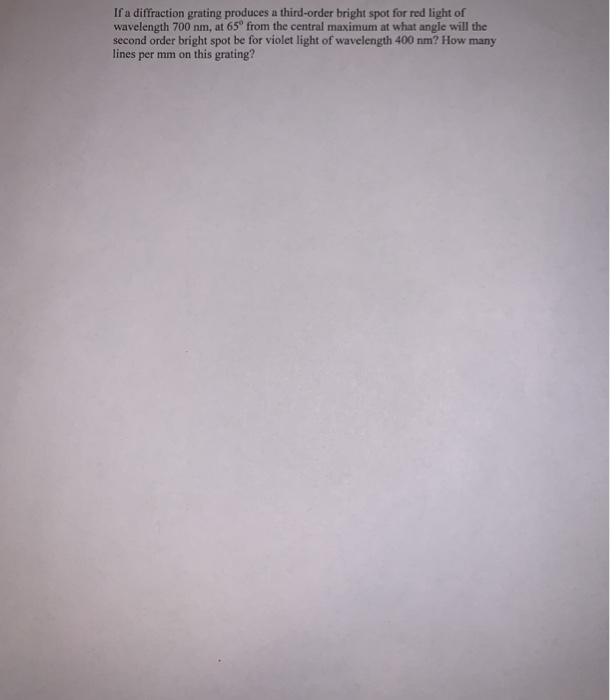If a diffraction grating produces a third-order bright spot for red light of wavelength 700 nm, at 65° from the central maximum at what angle will the second order bright spot be for violet light of wavelength 400 nm? How many lines per mm on this grating? If a diffraction grating produces a third-order bright spot for red light of wavelength 700 nm, at 65° from the central maximum at what angle will the second order bright spot be for...

• ### A 480 lines/mm diffraction grating is illuminated by light of wavelength 510 nm . How many...

A 480 lines/mm diffraction grating is illuminated by light of wavelength 510 nm . How many bright fringes are seen on a 4.0-m-wide screen located 2.1 m behind the grating?

• ### A diffraction grating has 5500 lines/cm . When a beam of monochromatic light goes through it,...

A diffraction grating has 5500 lines/cm . When a beam of monochromatic light goes through it, the second pair of bright spots occurs at ±26.0 cm from the central spot on a screen 43.0 cm past the grating. A. What is the wavelength of this light? B. How far from the central spot does the next pair of bright spots occur on the screen?

• ### Question 3: Light of wavelength 500 nm falls upon a diffraction grating with 800 lines/mm. a)...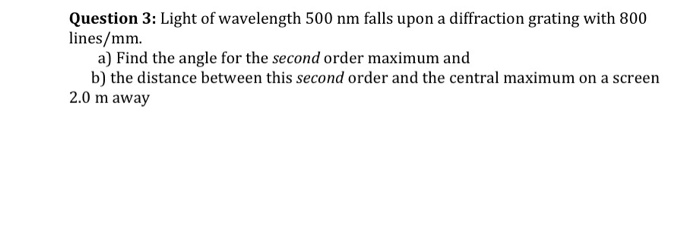Question 3: Light of wavelength 500 nm falls upon a diffraction grating with 800 lines/mm. a) Find the angle for the second order maximum and b) the distance between this second order and the central maximum on a screen 2.0 m away

• ### 1. Monochromatic blue light of wavelength 440-nm passes through a 3300 lines/cm diffraction grating and the...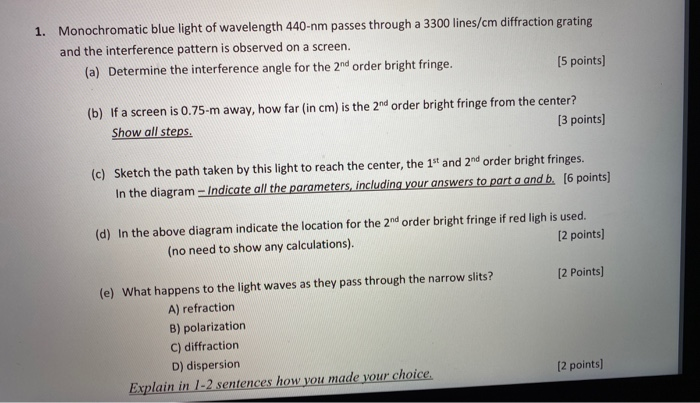1. Monochromatic blue light of wavelength 440-nm passes through a 3300 lines/cm diffraction grating and the interference pattern is observed on a screen. (a) Determine the interference angle for the 2nd order bright fringe. (5 points) (b) If a screen is 0.75-m away, how far (in cm) is the 2nd order bright fringe from the center? Show all steps. [3 points) (c) Sketch the path taken by this light to reach the center, the 1st and 2nd order bright fringes....

• ### 1. Monochromatic blue light of wavelength 440-nm passes through a 3300 lines/cm diffraction grating and the...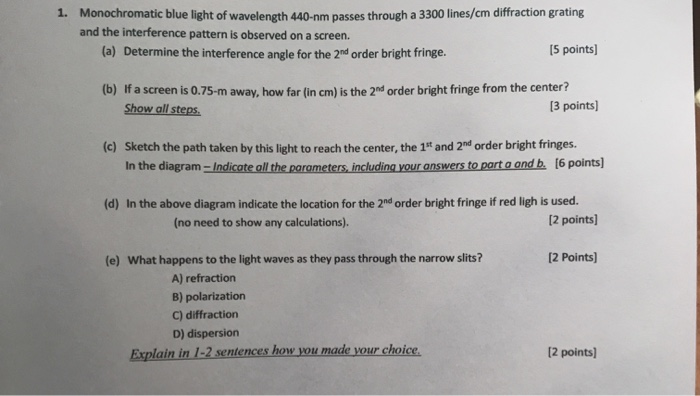1. Monochromatic blue light of wavelength 440-nm passes through a 3300 lines/cm diffraction grating and the interference pattern is observed on a screen. (a) Determine the interference angle for the 2nd order bright fringe. 15 points) (b) If a screen is 0.75-m away, how far (in cm) is the 2nd order bright fringe from the center? Show all steps. [3 points) (c) Sketch the path taken by this light to reach the center, the 1st and 2nd order bright fringes....

Free Homework App